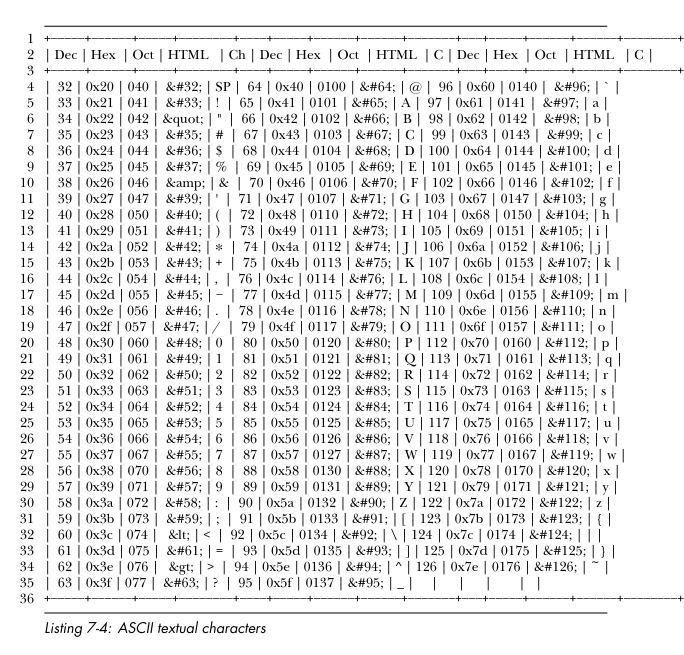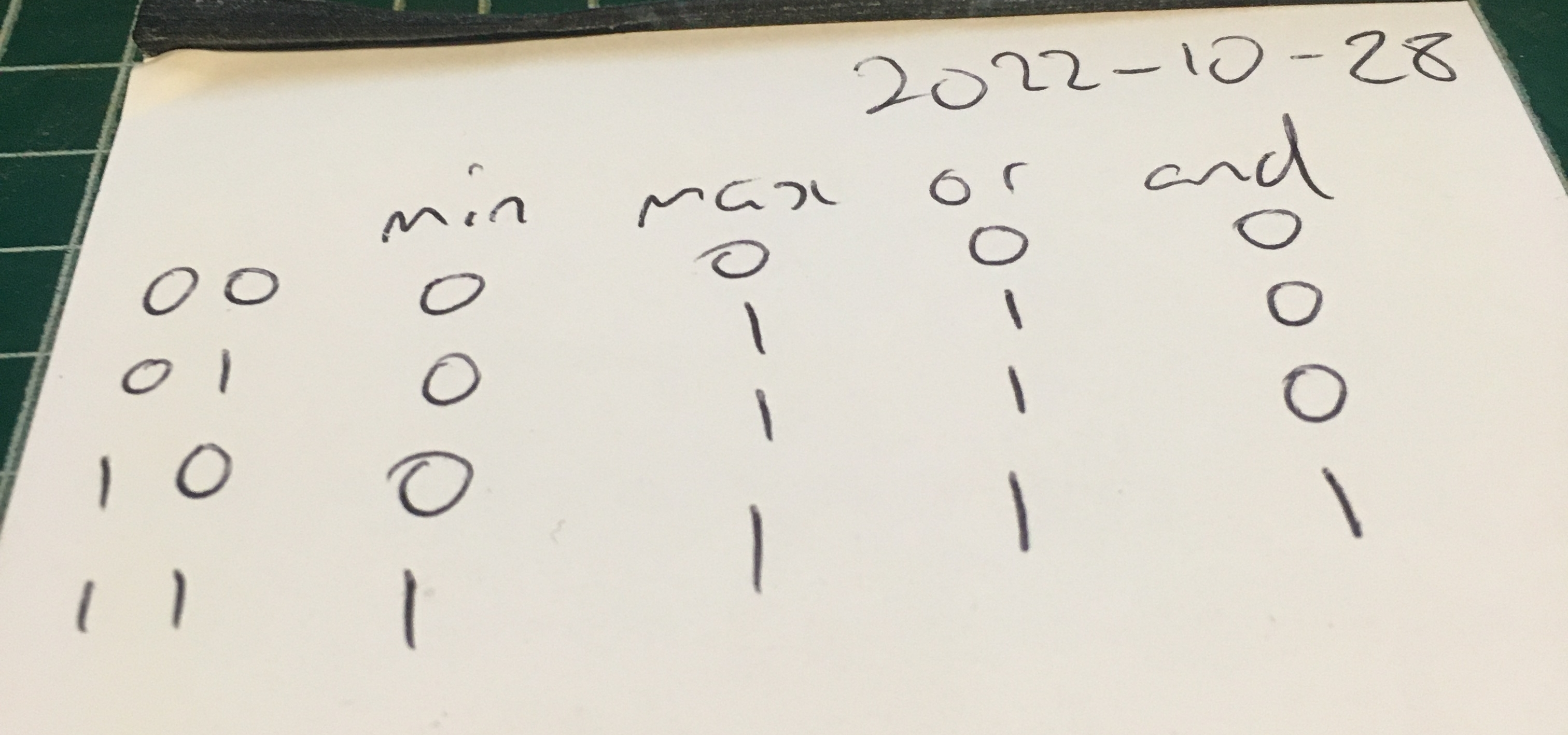# Botched ASCII table in LaTeX

Well this didn’t work. It was my first attempt at putting an ASCII chart into my book. (Because what the world needs is more books with ASCII tables in them!) I tried using the output of my ascii.php program. Tomorrow I will redo as vector graphics using Inkscape.# Boolean functions

Today I realised that ‘and’ == ‘minimum’ and ‘or’ == ‘maximum’. Mind blown! :)And here is some PHP code to go with my new found knowledge:

```test( 0, 0 );
test( 0, 1 );
test( 1, 0 );
test( 1, 1 );

function test( \$x, \$y ) {

if ( and_1( \$x, \$y ) !== and_2( \$x, \$y ) ) { echo "Error.\n"; }

if (  or_1( \$x, \$y ) !==  or_2( \$x, \$y ) ) { echo "Error.\n"; }

}

function and_1( \$x, \$y ) {

return (bool)min( \$x, \$y );

}

function and_2( \$x, \$y ) {

return ! ( ! \$x || ! \$y );

}

function or_1( \$x, \$y ) {

return (bool)max( \$x, \$y );

}

function or_2( \$x, \$y ) {

return ! ( ! \$x && ! \$y );

}```